• 复图像做相关
2022-07-06 09:51:27

## 1 内容简介

针对同一场景的多聚焦图像融合,提出了一种基于双树复小波变换(DT-CWT)的图像融合新算法.首先利用DT-CWT对图像进行多尺度和多方向分解,并根据双树复小波分解域各子带的系数特性定义了图像局部方向对比度,然后针对高频分量系数的选择,采用基于方向对比度的融合规则,而在低频域采用图像清晰度为测度的融合策略.实验结果表明,该算法能够很好地将多聚焦图像中的重要信息提取并注入到融合图像中,与其他方法相比较,取得了更好的融合效果,提高了融合图像的质量.

图像融合是信息(数据)融合的重要分支。图像融 合指通过对两幅或多幅图像(源图像)中具有互补性的 信息进行提取和综合 ‚获得对某一场景或目标更为准 确、全面、可靠的图像描述 ‚使融合图像更符合人或机器 的视觉特性 ‚以利于对图像的进一步分析和理解。图像 融合在自动目标识别、计算机视觉、遥感、医学图像处理 及军事等领域有着广泛的应用。多聚焦图像融合是 图像融合研究内容之一 ‚这一技术将相同的成像条件下 镜头聚焦目标不同的两个(或多个)原图像合成一幅多 目标都聚焦清晰的融合图像 ‚使之更适合人眼观察或计 算机的后续处理 。

基于小波变换的图像融合技术代表了当今像素级 多聚焦图像融合技术的发展前沿之一。 目前常用的离 散小波变换(Discrete Wavelet Transform ‚DWT )技术 虽然具有局部性 ‚多分辨率等优点 ‚但它仍然不是一个 让人完全满意的图像表达方式 ‚不仅会丧失傅里叶变换的平移不变性 ‚而且不能很好地表现图像中的方向特 征。然而双树复小波变换(Dual-Tree Complex Wave- let Transform ‚DT-CWT )却能克服这些不足 ‚它不仅 保持了传统小波优良的时频局部化分析能力 ‚还具有优 良的方向分析能力 ‚能够反映图像在不同分辨率上沿多 个方向变化的情形 ‚更好地描述图像的方向性。双树复 小波变换在图像去噪、边缘提取、纹理分析中都有很好 的表现 ‚近年来也被应用到图像融合领域。这里 基于双树复小波变换进行多聚焦图像的融合 ‚能够更好 地提取原图像中的方向性信息 ‚使融合图像的边缘和纹 理更加清晰 ‚所含信息量更加丰富。本文对高低频子带 采用不同的融合策略。在高频子带融合中引入相似性 度量 ‚在此基础上采用局部能量取大和加权平均相结合 的融合策略。实验结果证实了该算法的优越性。

## 2 参考代码

function y = icplxdual2D(w, J, Fsf, sf)

% Inverse Dual-Tree Complex 2D Discrete Wavelet Transform %  % USAGE: %   y = icplxdual2D(w, J, Fsf, sf) % INPUT: %   w - wavelet coefficients %   J - number of stages %   Fsf - synthesis filters for final stage %   sf - synthesis filters for preceeding stages % OUTPUT: %   y - output array % See cplxdual2D % % WAVELET SOFTWARE AT POLYTECHNIC UNIVERSITY, BROOKLYN, NY % http://taco.poly.edu/WaveletSoftware/

for j = 1:J     for m = 1:3         [w{j}{1}{1}{m} w{j}{2}{2}{m}] = pm(w{j}{1}{1}{m},w{j}{2}{2}{m});         [w{j}{1}{2}{m} w{j}{2}{1}{m}] = pm(w{j}{1}{2}{m},w{j}{2}{1}{m});     end end

y = zeros(size(w{1}{1}{1}{1})*2); for m = 1:2     for n = 1:2         lo = w{J+1}{m}{n};         for j = J:-1:2             lo = sfb2D(lo, w{j}{m}{n}, sf{m}, sf{n});         end         lo = sfb2D(lo, w{1}{m}{n}, Fsf{m}, Fsf{n});         y = y + lo;     end end

% normalization y = y/2;

function y = sfb2D_A(lo, hi, sf, d)

% 2D Synthesis Filter Bank % (along single dimension only) % % y = sfb2D_A(lo, hi, sf, d); % sf - synthesis filters % d  - dimension of filtering % see afb2D_A

lpf = sf(:, 1);     % lowpass filter hpf = sf(:, 2);     % highpass filter

if d == 2    lo = lo';    hi = hi'; end

N = 2*size(lo,1); L = length(sf); y = upfirdn(lo, lpf, 2, 1) + upfirdn(hi, hpf, 2, 1); y(1:L-2, :) = y(1:L-2, :) + y(N+[1:L-2], :); y = y(1:N, :); y = cshift2D(y, 1-L/2);

if d == 2    y = y'; end

function [af, sf] = dualfilt1

% Kingsbury Q-filters for the dual-tree complex DWT % % USAGE: %    [af, sf] = dualfilt1 % OUTPUT: %    af{i}, i = 1,2 - analysis filters for tree i %    sf{i}, i = 1,2 - synthesis filters for tree i %    note: af{2} is the reverse of af{1} % REFERENCE: %    N. G. Kingsbury,  "A dual-tree complex wavelet %    transform with improved orthogonality and symmetry %    properties", Proceedings of the IEEE Int. Conf. on %    Image Proc. (ICIP), 2000 % See dualtree % % WAVELET SOFTWARE AT POLYTECHNIC UNIVERSITY, BROOKLYN, NY % http://taco.poly.edu/WaveletSoftware/

% These cofficients are rounded to 8 decimal places.

af{1} = [    0.03516384000000                  0                   0                  0   -0.08832942000000  -0.11430184000000    0.23389032000000                  0    0.76027237000000   0.58751830000000    0.58751830000000  -0.76027237000000                   0   0.23389032000000   -0.11430184000000   0.08832942000000                   0                  0                   0  -0.03516384000000  ];   af{2} = [                   0  -0.03516384000000                   0                  0   -0.11430184000000   0.08832942000000                   0   0.23389032000000    0.58751830000000  -0.76027237000000    0.76027237000000   0.58751830000000    0.23389032000000                  0   -0.08832942000000  -0.11430184000000                   0                  0    0.03516384000000                  0 ];   sf{1} = af{1}(end:-1:1, :);   sf{2} = af{2}(end:-1:1, :);

% Dual tree complex wavelet transform (DT-CWT) based image fusion demo
% By VPS Naidu, MSDF Lab, June 2011
% DT-CWT software used in this fusion algorithm is from
clear all; close all; home;

% User selection (1,2,3,...)
J = 6; % number of decomposition levels used in the fusion

[Faf,Fsf] = FSfarras; % first stage filters
[af,sf] = dualfilt1;  % second stage filters

% images to be fused
figure; subplot(121);imshow(im1,[]); subplot(122); imshow(im2,[]);

% image decomposition
w1 = cplxdual2D(im1,J,Faf,af);
w2 = cplxdual2D(im2,J,Faf,af);

% Image fusion process start here
for j=1:J % number of stages
for p=1:2 %1:real part & 2: imaginary part
for d1=1:2 % orientations
for d2=1:3
x = w1{j}{p}{d1}{d2};
y = w2{j}{p}{d1}{d2};
D  = (abs(x)-abs(y)) >= 0;
wf{j}{p}{d1}{d2} = D.*x + (~D).*y; % image fusion
end
end
end
end
for m=1:2 % lowpass subbands
for n=1:2
wf{J+1}{m}{n} = 0.5*(w1{J+1}{m}{n}+w2{J+1}{m}{n}); % fusion of lopass subbands
end
end

% fused image
imf = icplxdual2D(wf,J,Fsf,sf);
figure; imshow(imf,[]);

​​​​​​​

## 3 运行结果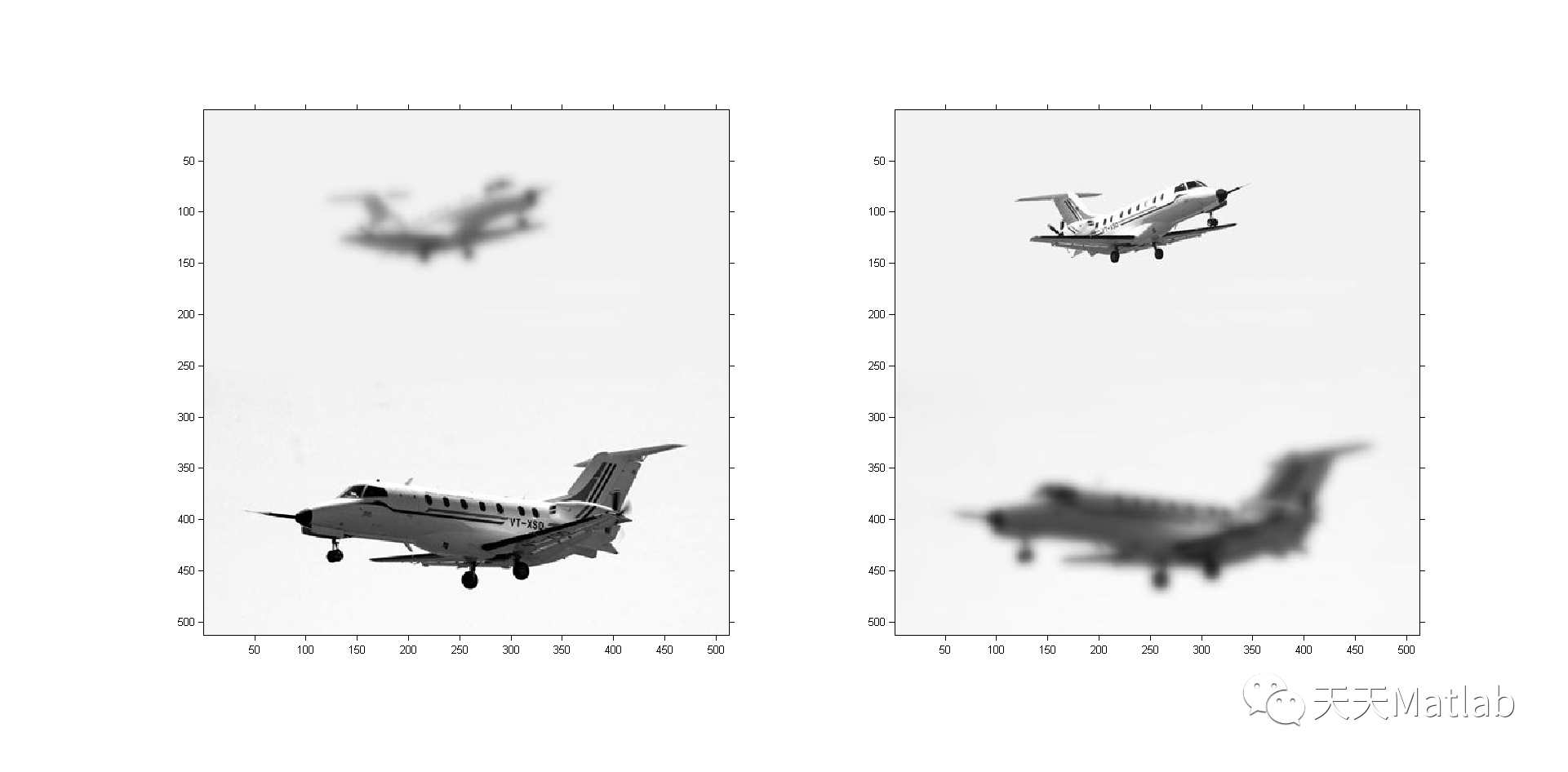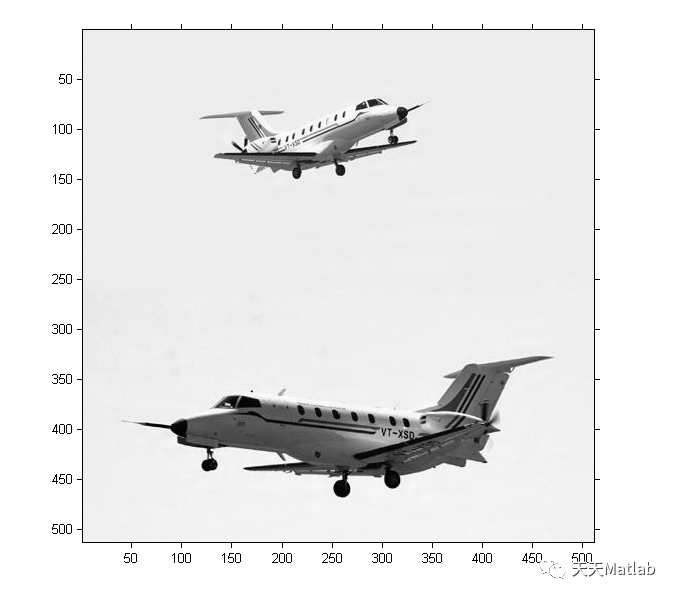## 4 参考文献

杨伟, 柴奇, 王黎明. 基于双树复小波变换的多聚焦图像融合方法[J]. 光电技术应用, 2009, 24(3):4.

### 博主简介：擅长智能优化算法、神经网络预测、信号处理、元胞自动机、图像处理、路径规划、无人机等多种领域的Matlab仿真，相关matlab代码问题可私信交流。

部分理论引用网络文献，若有侵权联系博主删除。算法 matlab 计算机视觉
更多相关内容
• 今天小编就为大家分享一篇python/Matplotlib绘制变函数图像教程，具有很好的参考价值，希望对大家有所帮助。一起跟随小编过来看看吧
• 研究了不同嵌入权重因子ω下藏有信息的宿主图像和解码后隐藏图像的各自相关度, 分析了在ω=0.2情况下藏有信息的宿主图像的剪切对提取的隐藏图像质量的影响和基于数字全息的三维物体信息隐藏, 结果表明该图像隐藏技术...
• 求两幅图像间的相关系数matlab的M文件---CorrelationCoefficient.m---；很简单的东东，但可以为你省些时间。共享改变未来！
• 为了使图像水印算法拥有更好的鲁棒性和不可感知性，以双树小波变换（DTCWT）为基础，提出了一种新的彩色图像盲水印算法。本算法将彩色图像颜色空间由RGB转换到YIQ，选取亮度分量Y进行双树小波变换，得到低频子带...
• 将二元树小波变换用于不同传感器图像的融合,对来自不同传感器图像进行Dual-Tree CWT分解,得到2个低频子图和6个高频子图,将低频部分进行加权平均,高频部分采用最大值选取法进行融合。对融合结果的性能采用熵、均...
• 基于IHS对偶树小波变换的遥感图像融合方法，高卫平，汪西莉，本文提出了基于对偶树小波变换(DT_CWT)和IHS变换结合的遥感图像融合算法，算法首先对多光谱图像进行IHS变换得到I分量，然后对IKONOS全
• ## 复指数信号e^jwt的图像及性质

千次阅读 多人点赞 2021-10-26 15:59:16
信号与系统、数字信号处理、信号分析，都离不开傅里叶变换，而傅里叶变换又离不开e^jwt。...关于e^jwt，好像很熟悉，很了解，但问你它是什么一个东西、有什么特点、相关的性质属性，你能马上说出来吗？ 首先， ...

信号与系统、数字信号处理、信号分析，都离不开傅里叶变换，而傅里叶变换又离不开 e j w t e^{jwt}
关于 e j w t e^{jwt} ，好像很熟悉，很了解，但问你它是什么一个东西、有什么特点、相关的性质属性，你能马上说出来吗？

首先， e j w t e^{jwt} 中j是虚数单位，w一般当做常量，t为变量。
关于虚数单位，应当回想起复数有关的知识，复数一般形如a+bj，可以在复平面用一根线表示，线的长度表示复数的模，与实轴（横轴）的夹角表示复数的相位。
既然 e j w t e^{jwt} 有自变量t（正是因为有了时间变量， e j w t e^{jwt} 也叫复指数信号），那应当可以画出它的图像。
谈到 e j w t e^{jwt} 的图像，你脑海里浮现出什么？
我脑海里竟想不出它的样子。
anyway，而今迈步从头越。

w=1;
t=0:0.1:20;
f=exp(1j*w*t);%语法问题，写代码最好用1j代替j
x=t;
y=imag(f);
z=real(f);
plot3(x,y,z,'.b');
xlabel('时间t');
ylabel('虚部y');
zlabel('实部z');


于是得到了 e j w t e^{jwt} 的图像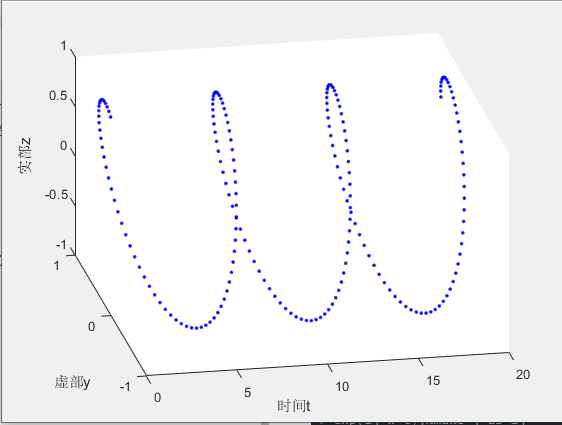（注意上图中的x坐标的方向，与一般我们稿纸画图的方向不同，看大家的图都不注明自变量的轴是哪个，难怪看晕了）

可以这样理解，画图本来就是先让自变量取一些值，然后在坐标系中描点，最后连线。
上图中的每一个点就是t取t0时， e j w t 0 e^{jwt0} 对应的值，由欧拉公式可知 e j w t 0 e^{jwt0} =cos(wt0)+jsin(wt0),得到的函数值是复数，复数要表示出来得需要两个坐标轴，这也是为什么画出来是三维的。

然后对这幅图进行解析，也就是探究它的性质。
首先，将所有的点在yoz平面上投影，得到的会是一个圆形。这是因为 e j w t e^{jwt} =cos(wt)+jsin(wt)，实部和虚部的平方和为1，所以 e j w t e^{jwt} 在复平面上表示就是一个单位圆。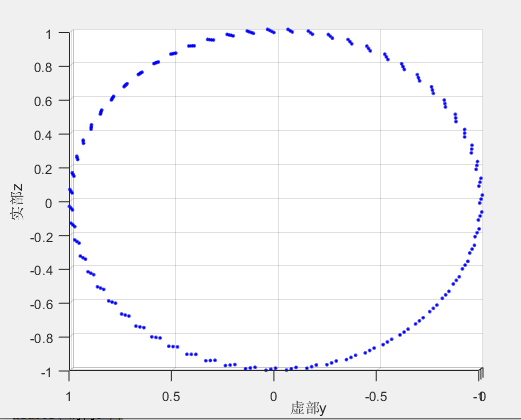（matlab画出来的三维图是可以拖曳从不同角度观察的，这是我拖到一个从x轴看过去的角度）

其次，关于w的物理意义和性质，上面那副图可以看着一个不断螺旋前进的轨迹图，w即反应了旋转的快慢。

最后，关于 e j w t e^{jwt} 。还可以看做一个单位长度，逆时针旋转的复平面旋转向量。展开全文数字信号处理
• 参照matlab绘制变函数的例子，使用python实现绘制变函数图像， ''' 参照matlab绘制变函数的例子，创建函数cplxgrid,cplxmap,cplxroot ''' # 1.导入相关库 import numpy as np import matplotlib.pyplot as ...

2018-09-15更新
今天发现sympy依赖的库mpmath里也有很多数学函数，其中也有在复平面绘制二维图的函数cplot，具体例子如下

from mpmath import *

def f1(z):
return z

def f2(z):
return z**3

def f3(z):
return (z**4-1)**(1/4)

def f4(z):
return 1/z

def f5(z):
return atan(z)

def f6(z):
return sqrt(z)

cplot(f1)
cplot(f2)
cplot(f3)
cplot(f4)
cplot(f5)
cplot(f6)

##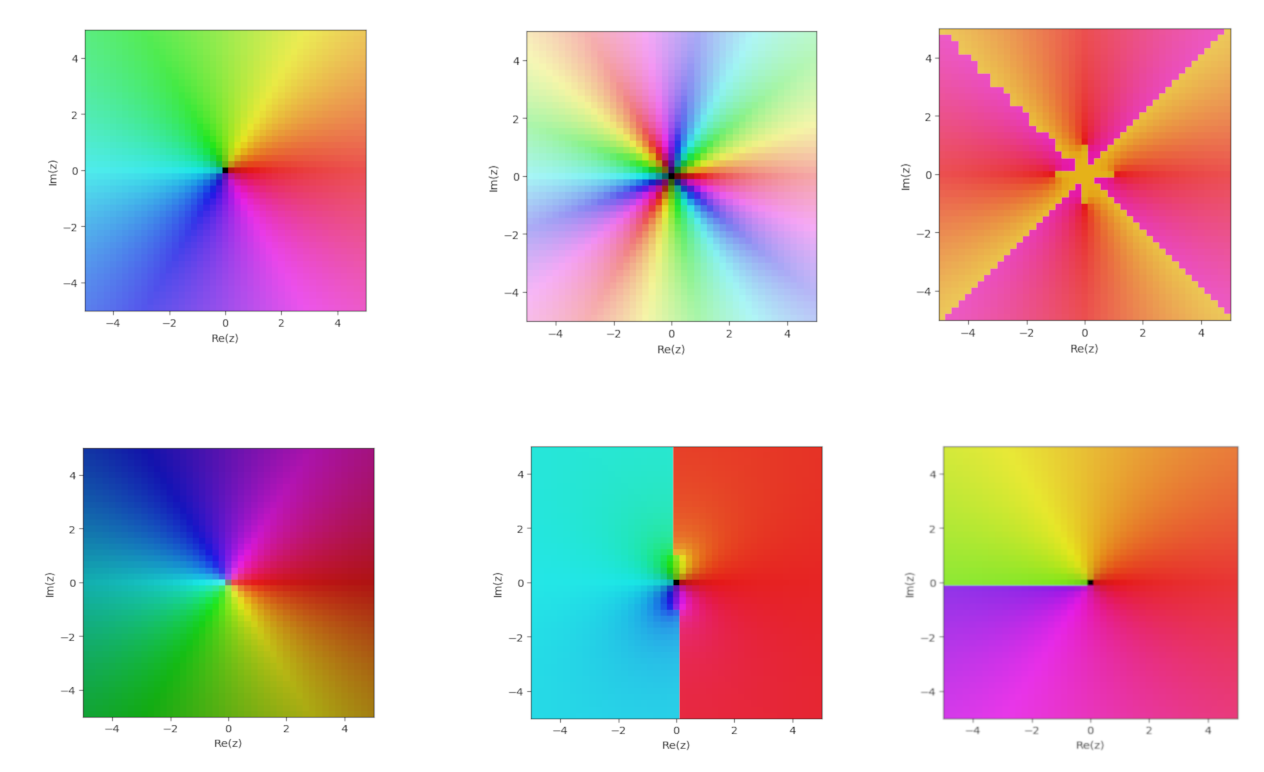参照matlab绘制复变函数的例子，使用python实现绘制复变函数图像，网上还没搜到相关的文章，在这里分享出来供大家学习。

'''
参照matlab绘制复变函数的例子，创建函数cplxgrid,cplxmap,cplxroot
'''
# 1.导入相关库
import numpy as np
import matplotlib.pyplot as plt
from mpl_toolkits.mplot3d import *

# 2.创建函数
def cplxgrid(m):
'''Return polar coordinate complex grid.

Parameters
----------
m: int

Returns
----------
z: ndarray,with shape (m+1)-by-(2*(m+1))
'''
m = m
r = np.arange(0,m).reshape(m,1) / m
theta = np.pi * np.arange(-m,m) / m
z = r * np.exp(1j * theta)

return z

def cplxroot(n=3,m=20):
'''
cplxroot(n): renders the Riemann surface for the n-th root
cplxroot(): renders the Riemann surface for the cube root.
cplxroot(n,m): uses an m-by-m grid.  Default m = 20.

Use polar coordinates, (r,theta).
Use polar coordinates, (r,theta).

Parameters
----------
n: n-th root
m: int

Returns
----------
None: Plot the Riemann surface
'''
m = m+1
r = np.arange(0,m).reshape(m,1) / m
theta = np.pi * np.arange(-n * m, n * m) / m
z = r * np.exp(1j * theta)
s = r * (1/n) * np.exp(1j * theta / n)
fig = plt.figure()
# ax.plot_surface(np.real(z),np.imag(z),np.real(s),color = np.imag(s))
ax.plot_surface(np.real(z),np.imag(z),np.real(s),cmap = plt.cm.hsv)
ax.set_xlim((-1,1))
ax.set_ylim((-1,1))
ax.set_xlabel('Real')
ax.set_ylabel('Imag')
ax.set_xticks([])
ax.set_yticks([])
ax.set_zticks([])
ax.set_autoscalez_on(True)#z轴自动缩放
ax.grid('on')
plt.show()

def cplxmap(z,cfun):
'''
Plot a function of a complex variable.

Parameters
----------
z: complex plane
cfun: complex function to plot

Returns
----------
None: Plot the surface of complex function
'''
blue = 0.2
x = np.real(z)
y = np.imag(z)
u = np.real(cfun)
v = np.imag(cfun)
M = np.max(np.max(u))#复变函数实部最大值
m = np.min(np.min(u))#复变函数实部最大值
s = np.ones(z.shape)
fig = plt.figure()
# 投影部分用线框图
surf1 = ax.plot_wireframe(x,y,m*s,cmap=plt.cm.hsv)
surf2 = ax.plot_surface(x,y,u,cmap=plt.cm.hsv)

#绘制复变函数1/z时会出错，ValueError: Axis limits cannot be NaN or Inf
# ax.set_zlim(m, M)
ax.set_xlim((-1,1))
ax.set_ylim((-1,1))
ax.set_xlabel('Real')
ax.set_ylabel('Imag')
ax.set_xticks([])
ax.set_yticks([])
ax.set_zticks([])
ax.set_autoscalez_on(True)#z轴自动缩放

ax.grid('on')
plt.show()

def _test_cplxmap():
'''测试cplxmap函数'''
z = cplxgrid(30)
w1 = z
w2 = z**3
w3 = (z**4-1)**(1/4)
w4 = 1/z
w5 = np.arctan(2*z)
w6 = np.sqrt(z)
w = [w1,w2,w3,w4,w5,w6]
for i in w:
cplxmap(z,i)

def _test_cplxroot():
'''测试cplxroot函数'''
cplxroot(n=2)
cplxroot(n=3)
cplxroot(n=4)
cplxroot(n=5)

if __name__ == '__main__':
_test_cplxmap()
_test_cplxroot()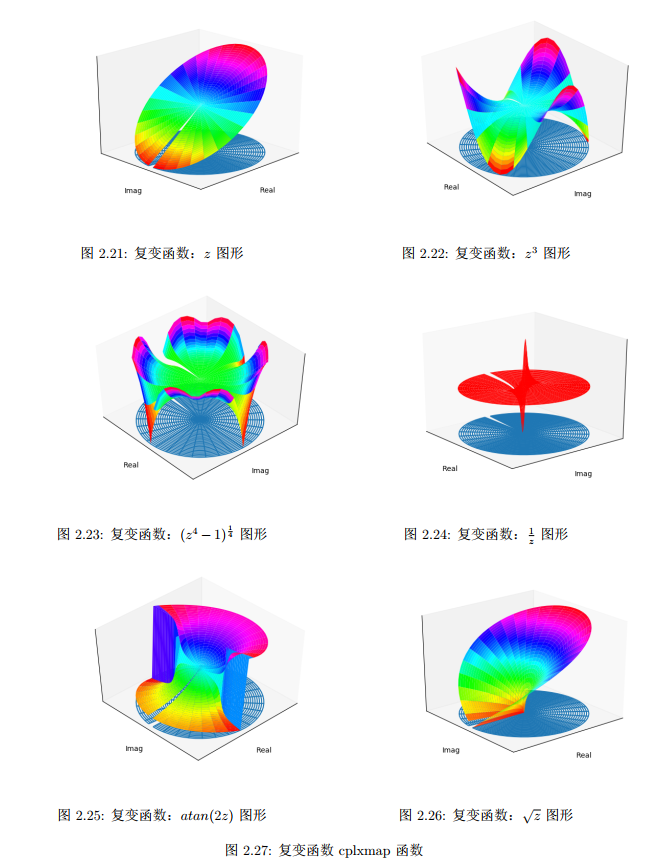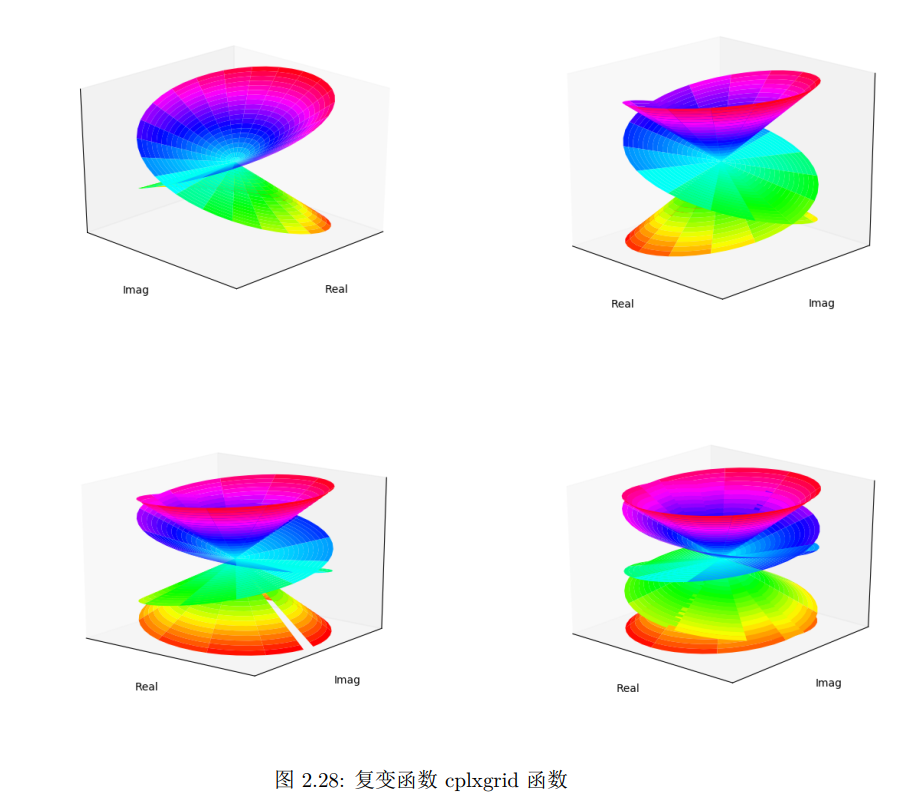展开全文python
• 音视频-编解码-目标图像的光学相关识别与运动模糊图像恢复.pdf
• 目录图像预处理一、颜色空间二、图像处理方法三、特征提取方法四、自适应直方图均衡五、CLAHE六、形态学运算七、空间域处理及其变换八、高斯金字塔九、拉普拉斯金字塔十、频率域分析——傅里叶变换十一、傅里叶变换...

# 图像预处理

## 一、颜色空间

1.RGB颜色空间
2.CMY(K)颜色空间
3.HSV颜色空间
4.CIE-XYZ颜色空间

## 二、图像处理方法## 三、特征提取方法直方图均衡化

直方图均衡化是指：利用图像直方图对对比度进行调整的方法
直方图均衡化通常用来增加许多图像的局部对比度，尤其是当图像的有用数据的对比度相当接近的时候。
直方图均衡化以后，亮度可以更改地在直方图上分布。这样就可以用于增强局部的对比度而不影响整体的对比度，直方图均衡化通过有效地扩展常用的亮度来实现这种功能。

## 四、自适应直方图均衡

直方图均衡的经典算法对整幅图像的像素使用相同的变换，如果图像中包括明显亮的或者暗的区域，则经典算法作用有限。
自适应直方图均衡（AHE）算法通过对局部区域进行直方图均衡，来解决上述问题。移动模板在原始图片上按特定步长滑动;
每次移动后，模板区域内做直方图均衡，映射后的结果赋值给模板区域内所有点，每个点会有多次赋值，最终的取值为这些赋值的均值。

## 五、CLAHE

AHE会过度放大图像中相对均匀区域的噪音，可采用限制对比度自适应直方图均衡（CLAHE）。与普通的自适应直方图均衡相比，CLAHE的不同地方在于直方图修剪过程，用修剪后的直方图均衡图像时，图像对比度会更自然。## 六、形态学运算

开运算∶先腐蚀再膨胀，可以去掉目标外的孤立点。
闭运算∶先膨胀再腐蚀，可以去掉目标内的孔。
通常，当有噪声的图像用阈值二值化后，所到的边界是很不平滑的，物体区域具有一些错判的孔洞，背景区域散布着一些小的噪声物体，连续的开和闭运算可以显著的改善这种情况。

## 七、空间域处理及其变换空间分析及变换空间分析及变换-平滑中值滤波/卷积空间分析及变换-平滑高斯滤波/卷积空间分析及变换-梯度Prewitt滤波/卷积空间分析及变换-梯度Sobel滤波/卷积空间分析及变换-梯度Laplacian滤波/卷积空间分析及变换-其他滤波/卷积## 八、高斯金字塔

图像金字塔化：先进行图像平滑，再进行降采样，根据降采样率，得到一系列尺寸逐渐减小的图像。操作：n次（高斯卷积->2倍降采样）->n层金字塔
目的：捕捉不同尺寸的物体图像缩放## 九、拉普拉斯金字塔

高频细节信息在卷积和下采样中丢失
保留所有层所丢失的高频信息，用于图像恢复## 十、频率域分析——傅里叶变换欧拉公式描述的是一个随着时间变化，在复平面上做圆周运动的点；傅里叶变换描述的就是一系列这样的点的运动叠加的效应离散傅里叶变换## 十一、傅里叶变换的不足

关键问题——傅里叶变换假设前提为信号平稳，但实际中信号多数为非平稳信号
缺乏时间和频率的定位功能；对于非平稳信号的局限性；在时间和频率分辨率上的局限性## 十二、短时傅里叶变换

STFT（短时傅里叶变换）添加时域信息的方法是设置窗格，认为窗格内的信号是平稳的；
然后对窗格内的信号分段进行傅里叶变换。
优点是可以获得频域信息的同时可以获得时域信息。
缺点是窗格大小很难设置。

短时傅里叶变换的特点
窄窗口时间分辨率高、频率分辨率低；宽窗口时间分辨率低，频率分辨率高。
对于时变的非稳态信号，高频适合小窗口，低频适合大窗口。可是STFT的窗口是固定的

## 十三、小波变换

小波变换与STFT思路接近，但小波变换直接把傅里叶变换的基给换了——将无限长的三角函数基换成了有限长的会衰减的小波基。
这样不仅能够获取频率，还可以定位到时间。常用的小波函数包括：
Haar系列，Daubechies系列，Moret系列
Sym系列，Meyer系列，Coif系列展开全文计算机视觉 opencv
• QSMART可根据复杂的MRI图像估算组织体积磁化率图。 QSMART是两阶段的QSM反转管线，可抑制伪影和静脉附近的斑纹伪影（图1）。 空间相关的滤波作为QSMART管道的一部分应用于组合的皮质表面和脉管系统蒙版，从而无需...
• OpenCV实现相位相关图像配准,编译产生库文件
• 该工具箱的目的是简化与图像分类相关的任务的常用计算机视觉功能（如HOG，SIFT，GIST和Color）的特征提取过程。 包含的功能的详细信息在中提供。 除了提供一些受欢迎的功能外，该工具箱还设计用于与不断增长的现代...
• 整理了近几十年图像增强相关论文，方便大家查看写国内外现状等等，祝大家论文多多！！！
• 目录 一、实验意义及目的 二、实验内容 ...（4）自行设计方法实现图像分割，并计算分割区域相关参数 一、实验意义及目的 （1）进一步掌握图像处理工具 Matlab，熟悉基于 Matlab 的图像处理函.matlab 计算机视觉
• 分别采用交叉有效性准则、修正复相关系数和 F 检验对回归方程的显著性进行检验，采用 Spearman 秩相关系数和平均相对误差对回归方程的预测性能进行检验。结果表明，建立的回归方程高度 显著，拟合度较好，预测性能...
•学习 计算机视觉
• 该算法可以解决有雾图像恢复时 天 空 区 域 的 颜 色 失 真 问 题，恢 图 像 的 细 节 和 颜 色，提 高 图 像 的 视 觉 效 果。 仿真结果表明，本文算法优于多尺度 Ｒｅｔｉｎｅｘ图像去雾算法。
• 针对数字图像相关方法中的亚像素插值误差，在频域内利用滤波器的传递函数分析了亚像素位置和图像频率对插值误差的影响，在此基础上设计了一种基于B样条插值核的优化插值滤波器。为使该滤波器能自适应于不同散斑...
• 立体图像质量是评价立体视频...在不同失真类型或混合失真评价结果中,Pearson线性相关系数(CC)值均在0.94以上,Spearman等级相关系数(SROCC)值均在0.92以上,符合人眼视觉特性,能够很好地预测人眼对立体图像的主观感知。
• 图像复原技术的主要目的是以预先确定的目标来改善图像，大部分是一个客观的过程。图像复原试图利用退化现象的某种先验知识来复原被退化的图像。因而，复原技术是面向退化模型的，并且采用相反的过程进行处理，以便...python
• 空域图像增强以及频域图像增强 | matlab图像处理2 提示：这里可以添加系列文章的所有文章的目录，目录需要自己手动添加 例如：第一章 Python 机器学习入门之pandas的使用 提示：写完文章后，目录可以自动生成，如何...matlab
• 提出一种重加权总变分与hyper-Laplacian相结合的图像盲复原算法。首先,通过重加权总变分先验重建模糊图像权重的双峰分布;然后,利用重建后的图像估计连续且稀疏分布的点扩展函数,并用其复原模糊图像,对以上两步反复...
• 电子科技大学图像与视频处理（图像部分）课后作业 作业2-1 1.已知一幅图像有8个灰度级，各个灰度级出现的概率如下表所示，画出该图像直方图，然后对该图像的直方图进行均衡化处理，并画出均衡化后的灰度直方图。 2...matlab...